# 2. Problem 3.16 in the text. Find and graph the even and odd parts of these...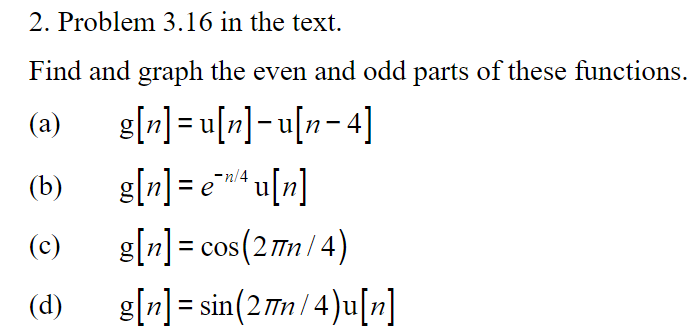2. Problem 3.16 in the text. Find and graph the even and odd parts of these functions. g[r] = u[n]=u[n=4] (a) g[n] = e"* u[n] -n/4 (b) g[n] = cos(2m /4) (c) g[r] = sin(2m/4)u (d)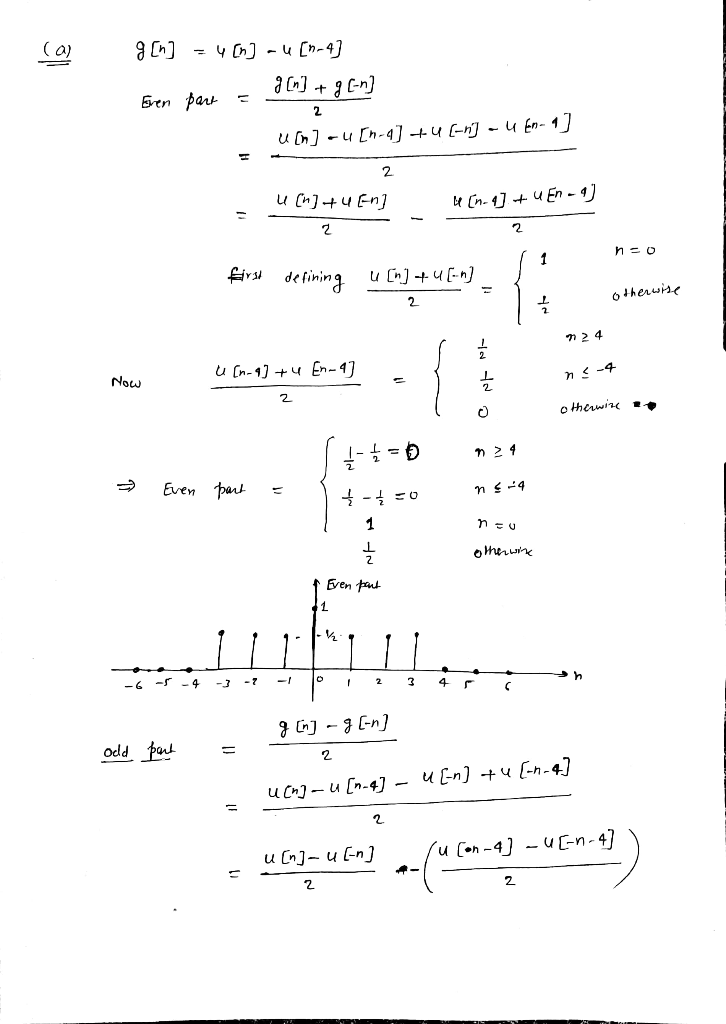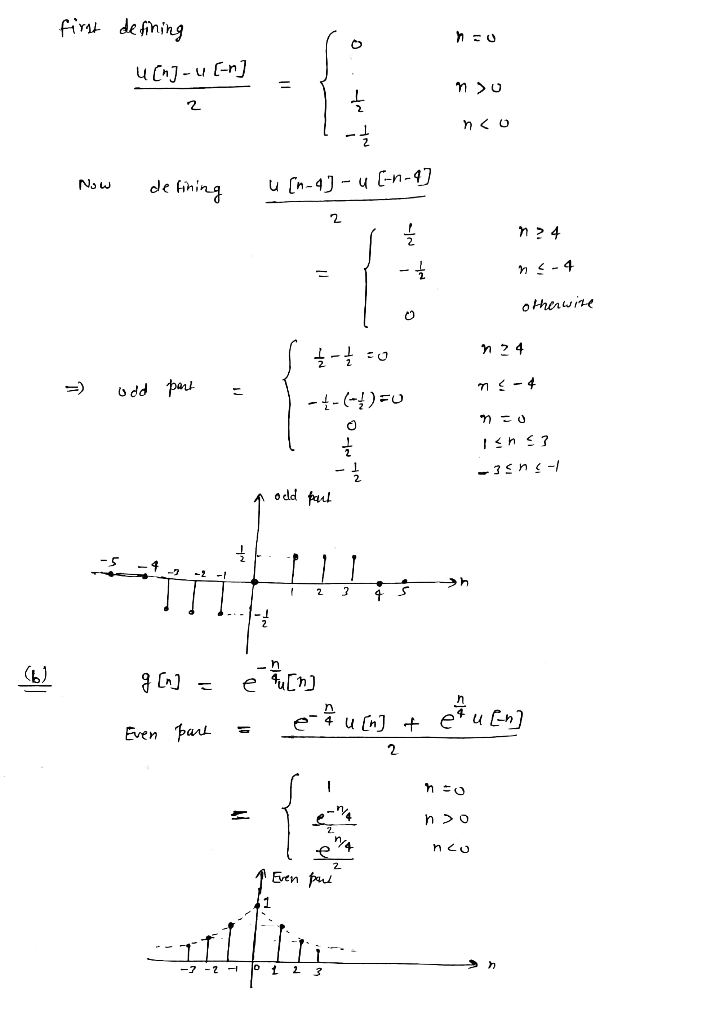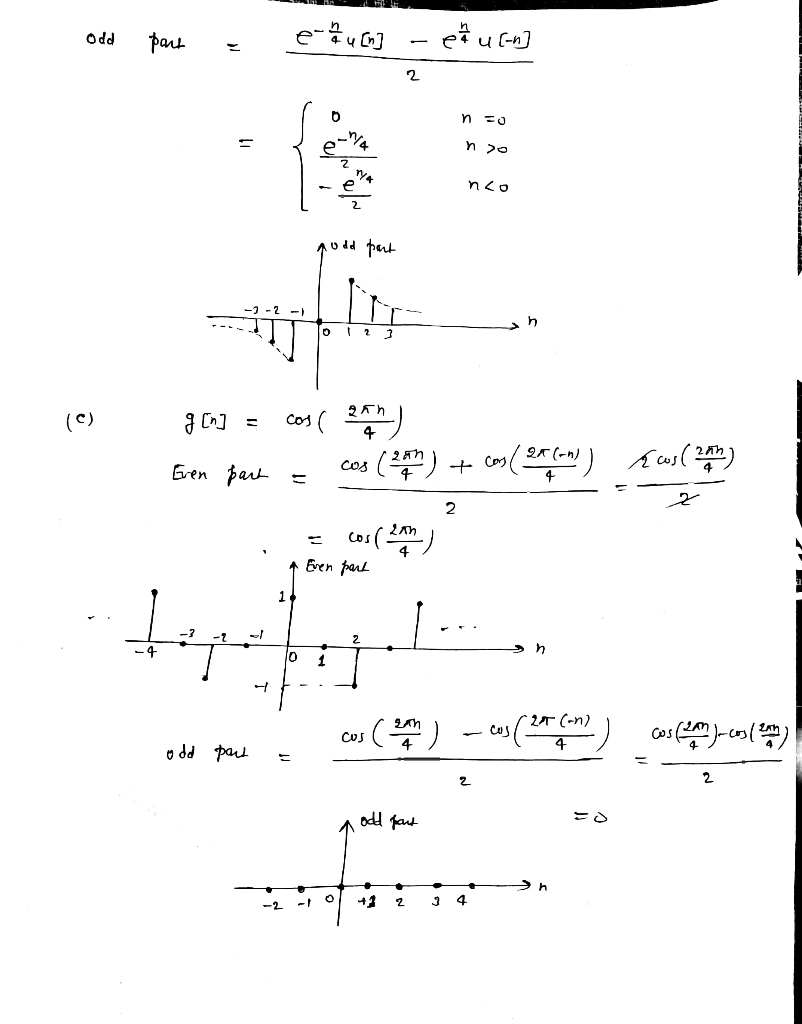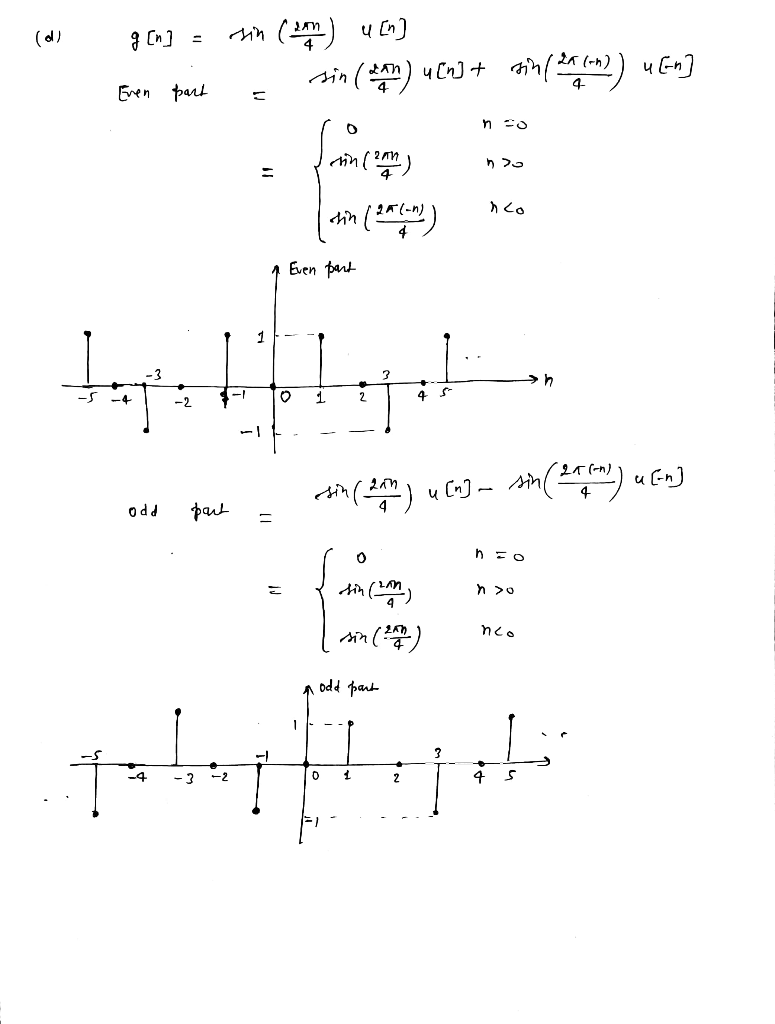#### Earn Coin

Coins can be redeemed for fabulous gifts.

Similar Homework Help Questions
• ### Find the even and odd parts of each of these CT functions 48. cos(Tt) 8 g(t)...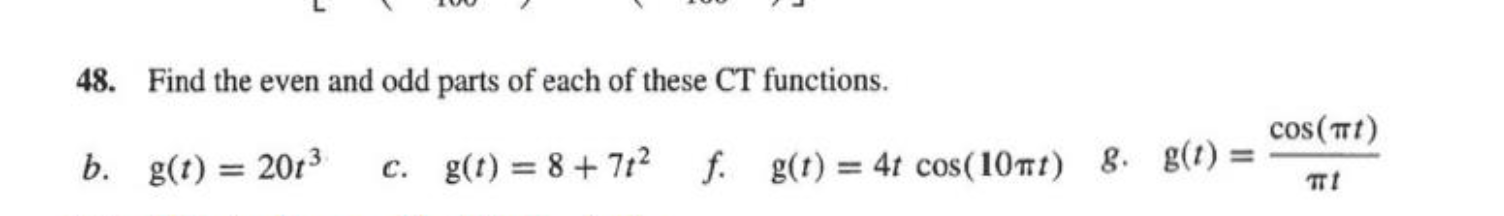Find the even and odd parts of each of these CT functions 48. cos(Tt) 8 g(t) : g(t)4t cos (10mt) g(t) 8+7t2f. TTt b. g(t) 20r3 C.

• ### Let n >k> 1 with n even and k odd. Make a k-regular graph G by putting n vertices in a circle and...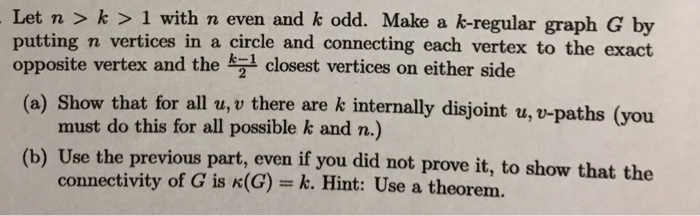need help with a and b in this graph theory question Let n >k> 1 with n even and k odd. Make a k-regular graph G by putting n vertices in a circle and connecting each vertex to the exact a) Show that for all u,v there are k internally disjoint u, v-paths (you (b) Use the previous part, even if you did not prove it, to show that the e vertex and the k 1 closest vertices on either...

• ### 1. Cousider the followving periodic function a) Determine whether the following function is odd, even or...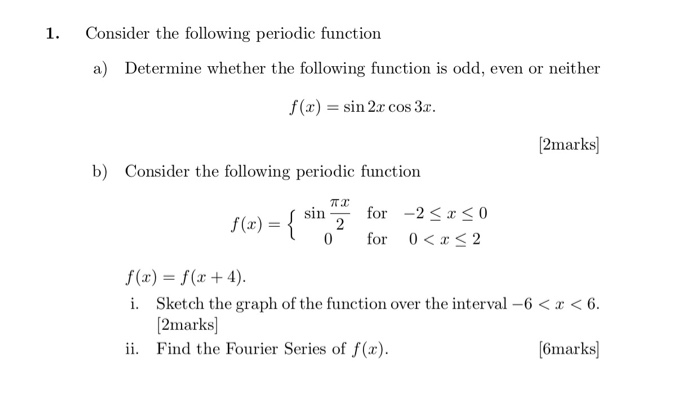1. Cousider the followving periodic function a) Determine whether the following function is odd, even or neither f(x) = sin 2x cos 3a. 2marks] Consider the following periodic function b) ㄫㄨ for -2 < x < 0 for 0< S 2 f(x) = { sin 0 f(x) = f(x + 4). i. Sketch the graph of the function over the interval-6< r <6. 2marks] Find the Fourier Series of f(x). (6marks ii.

• ### Odd and Even Functions An even function has the property f(x) =f(-x). Consider the function f(x)...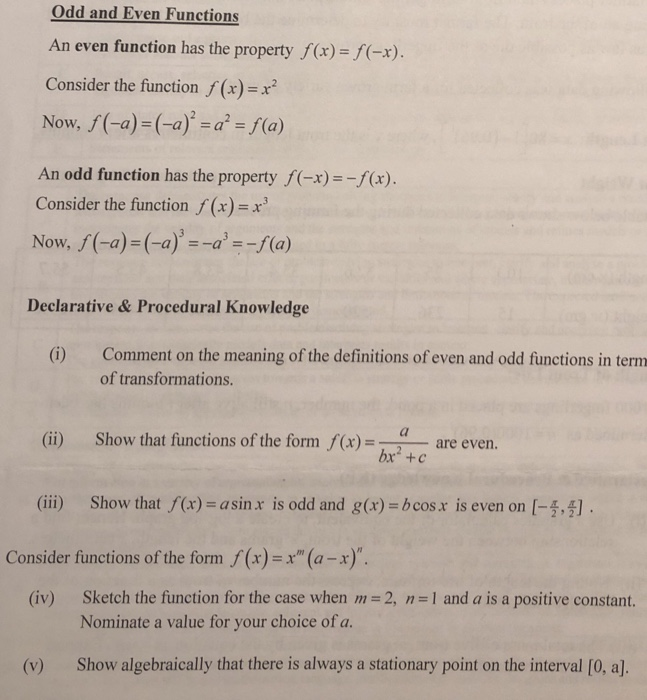Odd and Even Functions An even function has the property f(x) =f(-x). Consider the function f(x) Now, f (-a)-(-a)"-d f(a) An odd function has the property f(-x)-f(x). Consider the function f(x) Now, f (-a) = (-a)' =-a3 =-f(a) Declarative & Procedural Knowledge Comment on the meaning of the definitions of even and odd functions in term of transformations. (i) (ii) Show that functions of the formx) are even. bx2 +c Show, that f(x) = asin xis odd and g(x) =...

• ### Fourier transforms using Properties and Table 1·2(t) = tri(t), find X(w) w rect(w/uo), find x(t) 2....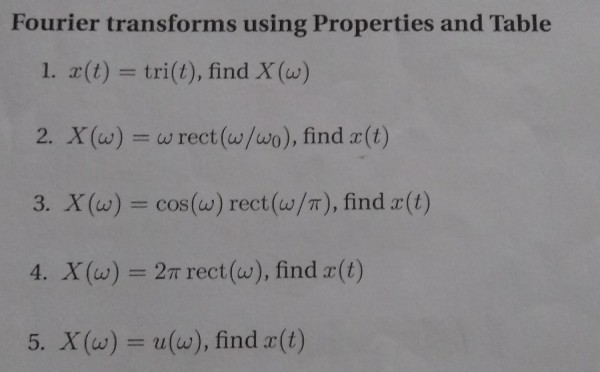Fourier transforms using Properties and Table 1·2(t) = tri(t), find X(w) w rect(w/uo), find x(t) 2. X(w) 3, x(w) = cos(w) rect(w/π), find 2(t) X(w)=2n rect(w), find 2(t) 4. 5, x(w)=u(w), find x(t) Reference Tables Constraints rect(t) δ(t) sinc(u/(2m)) elunt cos(wot) sin(wot) u(t) e-ofu(t) e-afu(t) e-at sin(wot)u(t) e-at cos(wot)u(t) Re(a) >0 Re(a) >0 and n EN n+1 n!/(a + ju) sinc(t/(2m) IIITo (t) -t2/2 2π rect(w) with 40 2r/T) 2Te x(u) = F {r) (u) aXi(u) +X2() with a E...

• ### Hollie work #2 (Due April 1 δ) Problem Obtain the Laplace transform of each of the...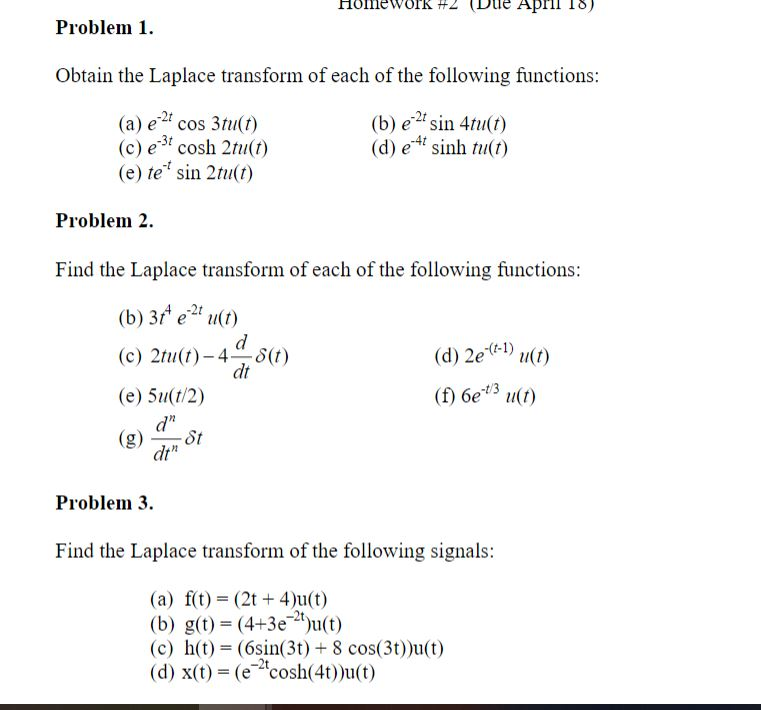Hollie work #2 (Due April 1 δ) Problem Obtain the Laplace transform of each of the following functions: 2t (a) et cos 3tu(t) (c) e3 cosh 2tu(t) (e) te sin 2tu(t) (b) e2t sin 4tu(t) (d) e4 sinh tu(t) Problem 2. Find the Laplace transform of each of the following functions (b) 3f* e^ut) (c) 2n1(t)-4". δ(t) (e) 5u(t/2) (d) 2e) u(t) 2p-(t-1) (f) 6el3 u(t) d" dt" Problem 3. Find the Laplace transform of the following signals (a) f(t)-(2t...

• ### Using the given graph of the function t, find whether the function is even, odd, or...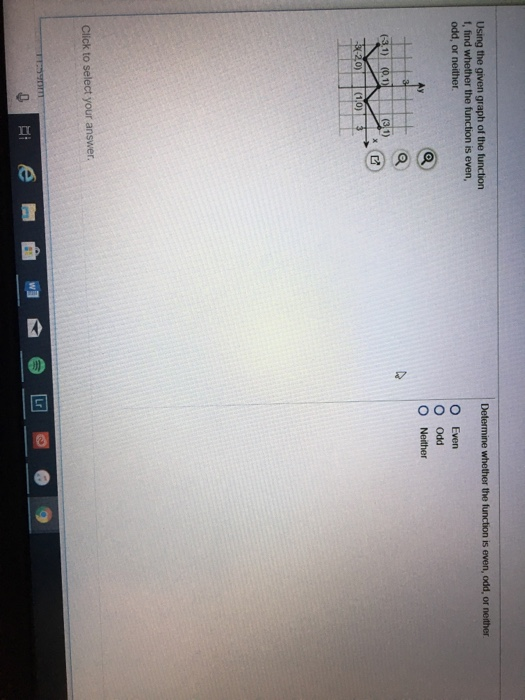Using the given graph of the function t, find whether the function is even, odd, or neither O Even O Odd O Neither 2 1) Click to select your answer 0

• ### Objective: • Graph and describe sinusoidal functions 1. Let x € R and let O be...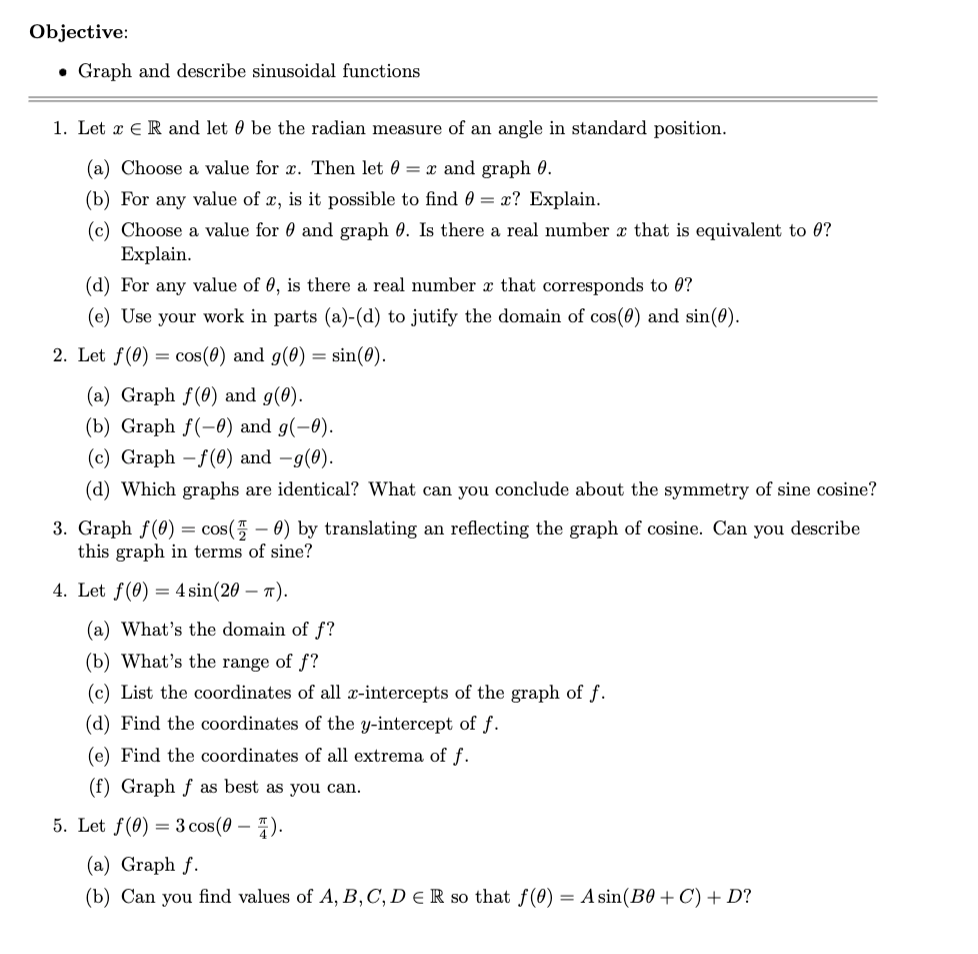Objective: • Graph and describe sinusoidal functions 1. Let x € R and let O be the radian measure of an angle in standard position. (a) Choose a value for z. Then let 0 = x and graph 0. (b) For any value of x, is it possible to find 0 = x? Explain. (c) Choose a value for 0 and graph 0. Is there a real number x that is equivalent to 0? Explain. (d) For any value of...

• ### Problem 4. Let V be the vector space of all infinitely differentiable functions f: [0, ]...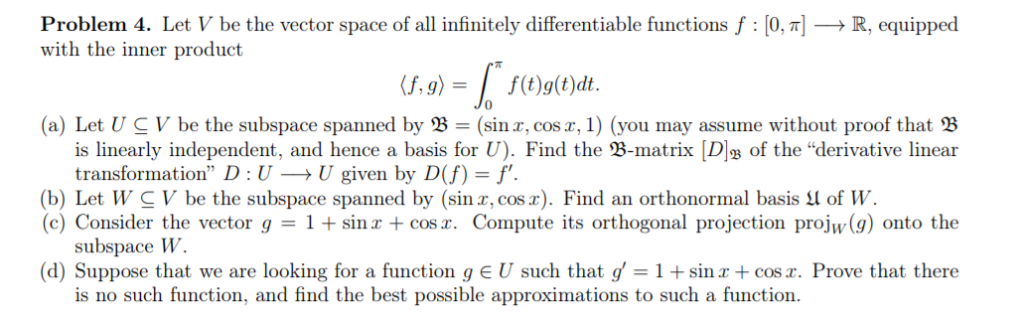Problem 4. Let V be the vector space of all infinitely differentiable functions f: [0, ] -» R, equipped with the inner product f(t)g(t)d (f,g) = (a) Let UC V be the subspace spanned by B = (sinr, cos x, 1) (you may assume without proof that B is linearly independent, and hence a basis for U). Find the B-matrix [D]93 of the "derivative linear transformation" D : U -> U given by D(f) = f'. (b) Let WC V...

• ### 5. Use a substitution and an integration by parts to find each of the following indef-...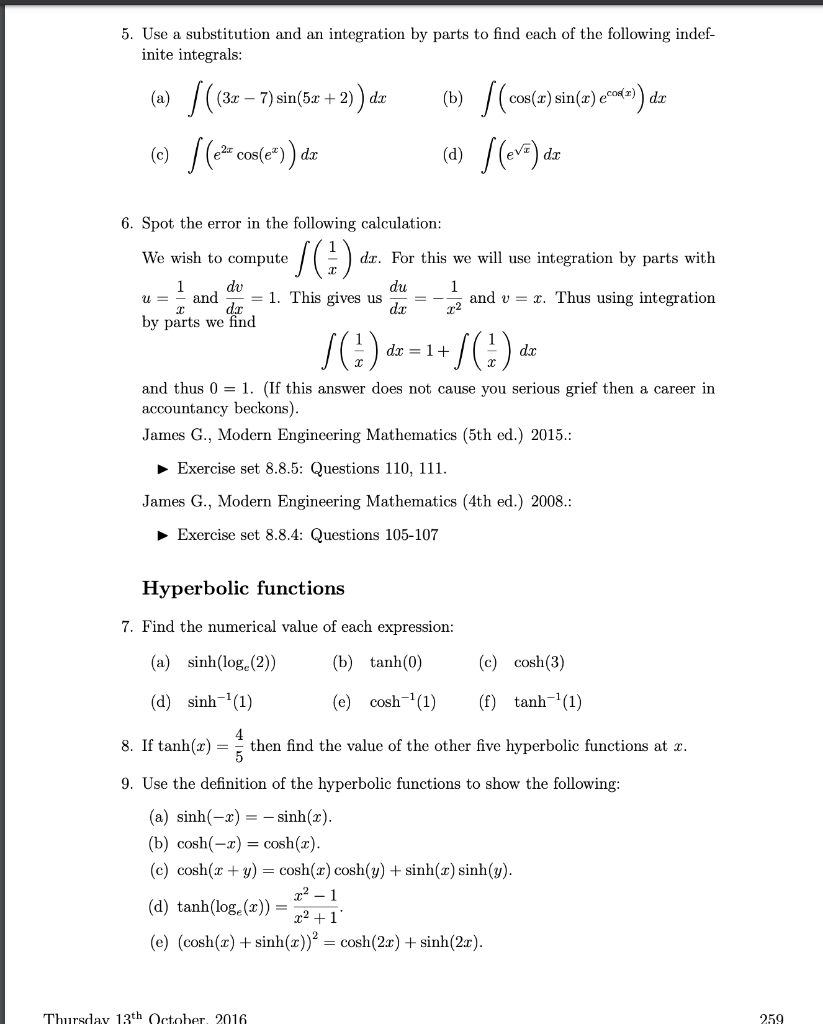5. Use a substitution and an integration by parts to find each of the following indef- inite integrals: (b) | (cos(a) sin(a) esas) de (a) / ( (32 – 7) sin(5x + 2)) de (c) / (e* cos(e=)) dt (d) dr 6. Spot the error in the following calculation: S() will use integration by parts with 1 We wish to compute dr. For this dv du 1 dar = 1. This gives us dr by parts we find dr =...